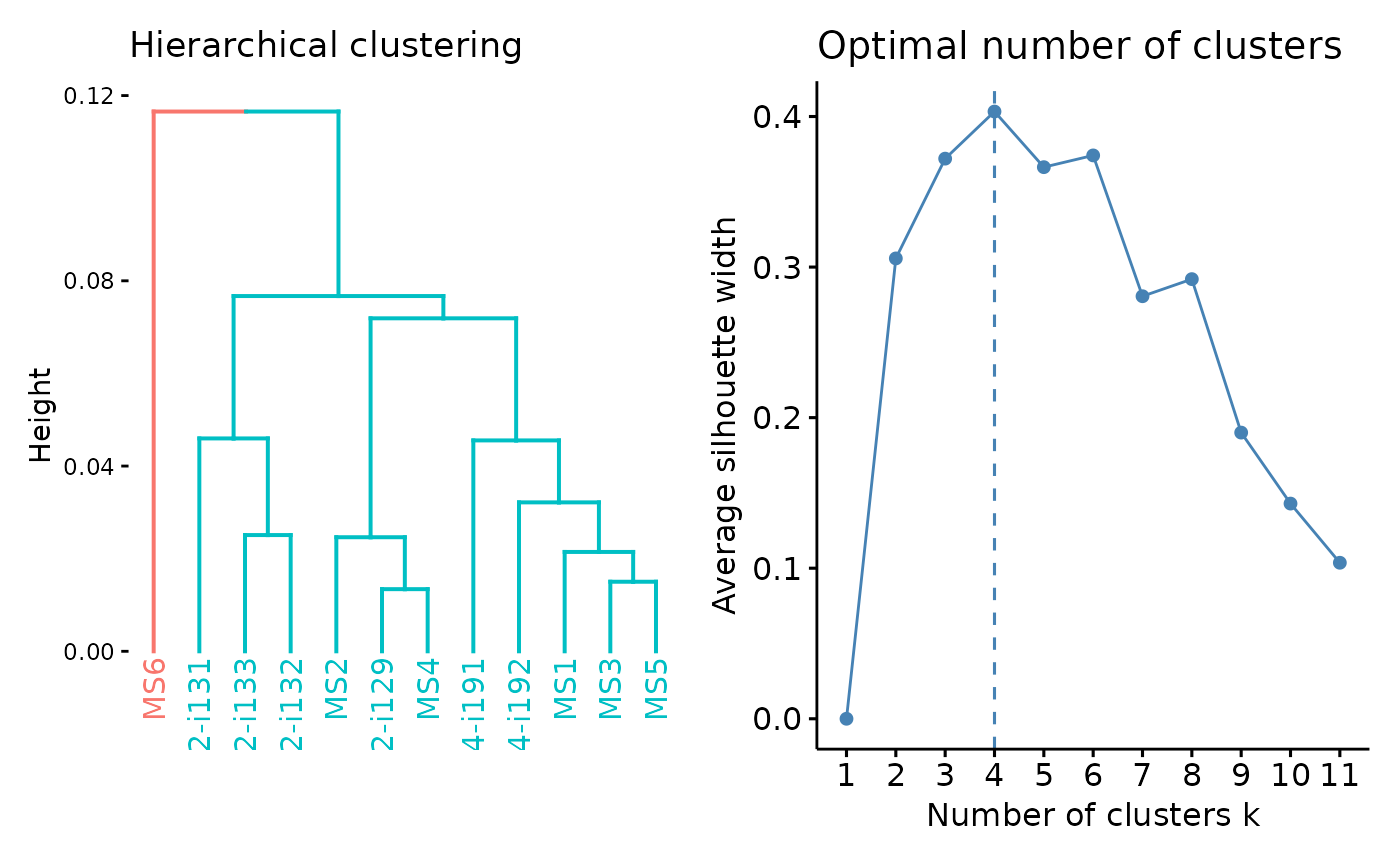The geneUsageAnalysis function deploys several data analysis methods, including PCA, multidimensional scaling, Jensen-Shannon divergence, k-means, hierarchical clustering, DBscan, and different correlation coefficients.

geneUsageAnalysis(
.data,
.method = c("js+hclust", "pca+kmeans", "anova", "js+pca+kmeans"),
.base = 2,
.norm.entropy = FALSE,
.cor = c("pearson", "kendall", "spearman"),
.do.norm = TRUE,
.laplace = 1e-12,
.verbose = TRUE,
.k = 2,
.eps = 0.01,
.perp = 1,
.theta = 0.1
)

## Arguments

.data

The geneUsageAnalysis function runs on the output from geneUsage.

.method

A string that defines the type of analysis to perform. Can be "pca", "mds", "js", "kmeans", "hclust", "dbscan" or "cor" if you want to calculate correlation coefficient. In the latter case you have to provide .cor argument.

.base

A numerical value that defines the logarithm base for Jensen-Shannon divergence.

.norm.entropy

A logical value. Set TRUE to normalise your data if you haven't done it already.

.cor

A string that defines the correlation coefficient for analysis. Can be "pearson", "kendall" or "spearman".

.do.norm

A logical value. If TRUE it forces Laplace smoothing, if NA it checks if smoothing is necessary, if FALSE does nothing.

.laplace

The numeric value, which is used as a pseudocount for Laplace smoothing.

.verbose

A logical value.

.k

The number of clusters to create, passed as k to hcut or as centers to kmeans.

.eps

A numerical value, DBscan epsylon parameter, see immunr_dbscan.

.perp

A numerical value, t-SNE perplexity, see immunr_tsne.

.theta

A numerical value, t-SNE theta parameter, see immunr_tsne.

## Value

Depends on the last element in the .method string. See immunr_tsne for more info.

## Examples

data(immdata)
gu <- geneUsage(immdata\$data, .norm = TRUE)
geneUsageAnalysis(gu, "js+hclust", .verbose = FALSE) %>% vis()
#> Warning: The <scale> argument of guides() cannot be FALSE. Use "none" instead as
#> of ggplot2 3.3.4.
#> ℹ The deprecated feature was likely used in the factoextra package.
#>   Please report the issue at <https://github.com/kassambara/factoextra/issues>.